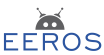#Real-Time Robotics Framework

### Site Tools

eeros_architecture:control_system:available_blocks:generic

# Basic Block

This is the basic block from which all other blocks inherit. But you can use the block directly and set its specific algorithm that will run whenever the block runs within a timedomain. Use the template parameters to choose any number of input and output signals together with their associated types. Choose this method when the algorithm is simple and one wants to avoid using several other blocks doing a simple algorithm, e.g. adding a offset and scale to a signal.

Define such a block with an example algorithm as follows:

  Blockio<2,1,Vector2,Vector2> block([&]() {
auto val = (block.getIn(0).getSignal().getValue() + 0.5) * 2;
val *= -1.0;
val += block.getIn(1).getSignal().getValue() + 1.0;
block.getOut().getSignal().setValue(val);
block.getOut().getSignal().setTimestamp(gen.getIn(0).getSignal().getTimestamp());
});

The algorithm can be passed to the constructor of the block with a lambda function. Such an algorithm could theoretically calculate any output from a given set of inputs. It can be especially valuable for cases like the following. A signal value must be applied with an offset and a scale. How to achieve this?

1. Use a constant block delivering the offset together with a sum block which adds the offset to the signal. Finally, a gain block applies the desired scale. All in all, you will use three different blocks.
2. Use a generic block and define the necessary algorithm as follows
GenericBlock<> gen([&]() {
gen.getOut(0).getSignal().setValue(gen.getIn(0).getSignal().getValue() + 0.1) * 1.1);
gen.getOut(0).getSignal().setTimestamp(gen.getIn(0).getSignal().getTimestamp());
})

Such a generic block can save valuable execution time in reducing the number of necessary blocks. It can also be useful when a given algorithm cannot be stitched together from predefined blocks.